# Graphing Derivatives & L'Hopital's Rule Flashcards

Graphing Derivatives & L'Hopital's Rule Flashcards
1/27 (missed) 0 0
Create Your Account To Continue Studying

As a member, you'll also get unlimited access to over 75,000 lessons in math, English, science, history, and more. Plus, get practice tests, quizzes, and personalized coaching to help you succeed.

Try it risk-free for 30 days. Cancel anytime.
###### Already registered? Login here for access
Determine the value of the derivative at x = -2 and x = 5, if the function has a maximum at x = -2 and a minimum at x = 5.
The derivative at both of the x-values is 0.
Got it
Given that the graph of the derivative of a function passes through the point (1, -3), make a statement about the original function.
At x = 1, the original function f(x) has a tangent line with a slope of -3.
Got it
At the point (-1, 4), the tangent line to f(x) has a slope of 7. Use this information to make a statement about a point on the graph of f '(x).
f '(x) contains the point (-1, 7)
Got it
Use the graph to answer the question below: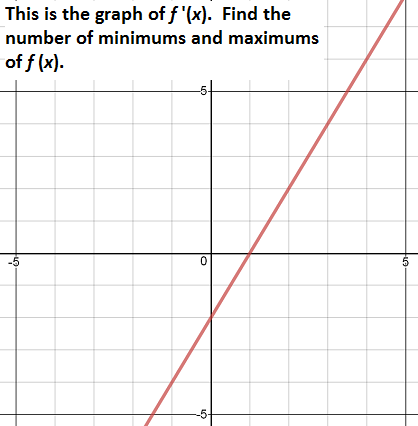f(x) has one minimum at x = 1.
Got it
Given the graph of f '(x), find f(x)f(x) = -1.5x2 + 6x + c, where c can be any constant
Got it
You are given that the slope of the tangent line to a particular function at x = 2 is 5. Determine the value of the derivative of that function at x = 2.
The derivative equals 5 at x = 2
Got it
List all x-values where f'(x) = 0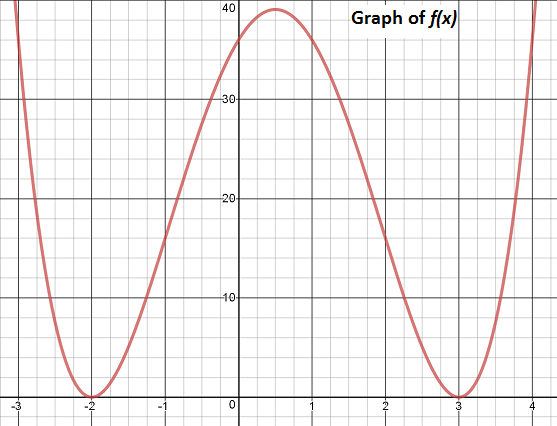f '(x) would equal zero at x = -2, 0.5, 3
Got it
Sketch the derivative of this function: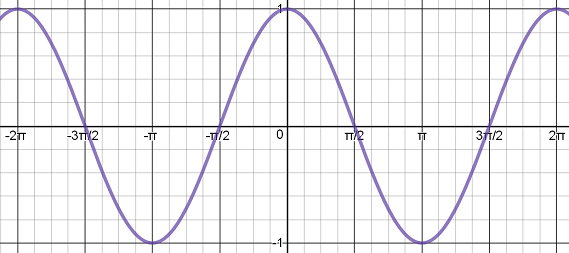The derivative should look like this: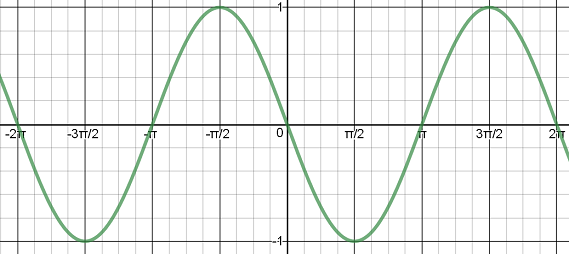Got it
Value of the derivative at a minimum or maximum of the function
At every x-value where the function has a minimum or a maximum, the derivative will equal 0.
Got it
Identify the curve of f(x) and that of f '(x).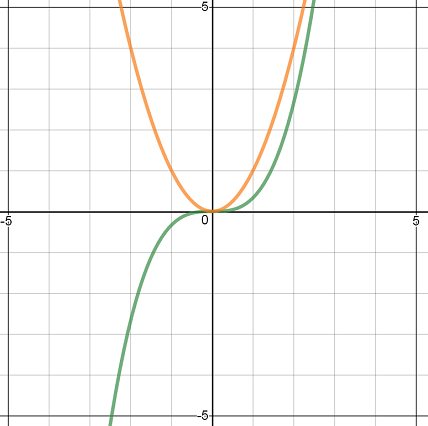The green curve is the function f(x) and the orange curve is its derivative f '(x).
Got it
Extremum (plural: extrema)
Any minimum or maximum values of a function
Got it
Find the limit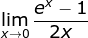The limit is 1/2
Got it
L'Hopital's Rule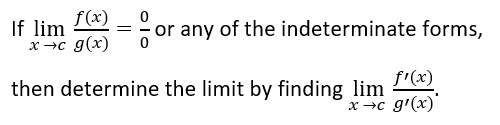Got it

### Ready to move on?

or choose a specific lesson: See all lessons in this chapter
27 cards in set

## Flashcard Content Overview

These flashcards contain practice problems for two different topics of calculus: Graphs of Derivatives and L'Hopital's Rule. You will be able to review how to use derivatives to determine extrema and concavity of functions. Additionally, you will be able to practice using derivatives to find limits by applying L'Hopital's Rule to a variety of functions.

Front
Back
L'Hopital's RuleFind the limitThe limit is 1/2
Extremum (plural: extrema)
Any minimum or maximum values of a function
Identify the curve of f(x) and that of f '(x).The green curve is the function f(x) and the orange curve is its derivative f '(x).
Value of the derivative at a minimum or maximum of the function
At every x-value where the function has a minimum or a maximum, the derivative will equal 0.
Sketch the derivative of this function:The derivative should look like this:List all x-values where f'(x) = 0f '(x) would equal zero at x = -2, 0.5, 3
You are given that the slope of the tangent line to a particular function at x = 2 is 5. Determine the value of the derivative of that function at x = 2.
The derivative equals 5 at x = 2
Given the graph of f '(x), find f(x)f(x) = -1.5x2 + 6x + c, where c can be any constant
Use the graph to answer the question below:f(x) has one minimum at x = 1.
At the point (-1, 4), the tangent line to f(x) has a slope of 7. Use this information to make a statement about a point on the graph of f '(x).
f '(x) contains the point (-1, 7)
Given that the graph of the derivative of a function passes through the point (1, -3), make a statement about the original function.
At x = 1, the original function f(x) has a tangent line with a slope of -3.
Determine the value of the derivative at x = -2 and x = 5, if the function has a maximum at x = -2 and a minimum at x = 5.
The derivative at both of the x-values is 0.
State the number of extrema of this function.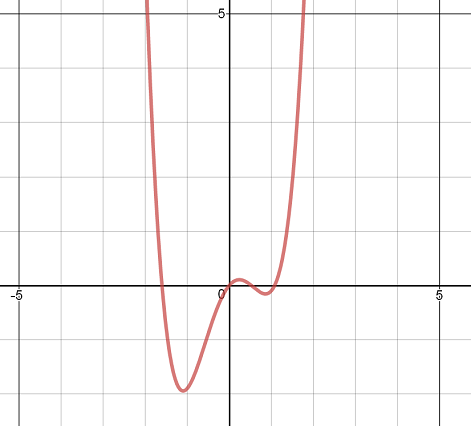This function has two minimums and one maximum.
Locate the function's maximum.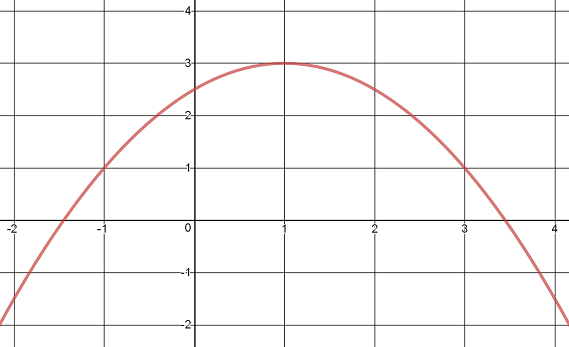The function has a maximum at x = 1.
State the location of the minimum.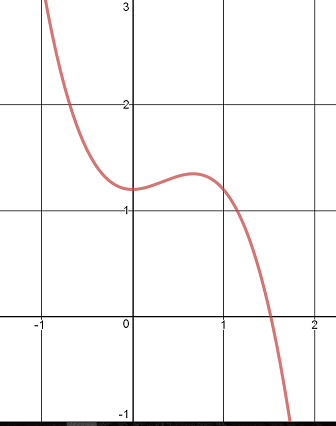The minimum of the function is at x = 0.
State the number of the extrema.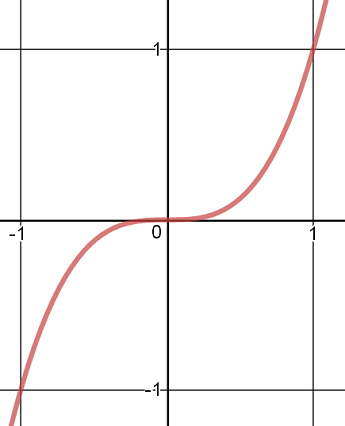This function has no minimums or maximums.
Find the limit: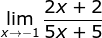The limit equals 2/5
Find the limit: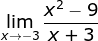The limit equals -6
Find the limit: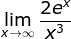The limit equals infinity
Find the limit: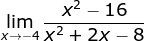The limit equals 4/3
Find the maximum value of f(x) = -x3 + 3x2 in the interval from x = 1 to x = 3.
The maximum value is 4
Determine the possible locations of extrema for y = 2x3 + 4x2 - 7
Possible extrema can be located at x = 0 and x = 4/3
Sketch the graph of a function such that the function is always positive, the first derivative is always positive, and the second derivative is always positive.
The graph should look like this: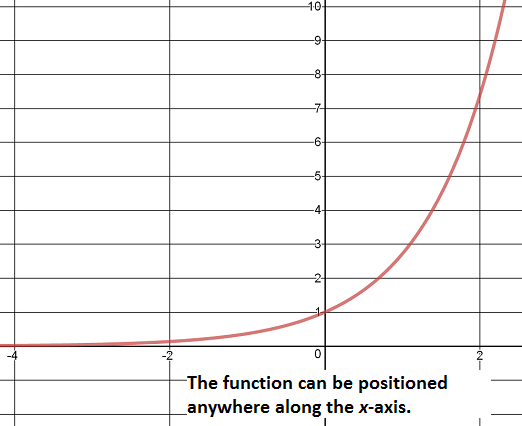Determine the value of a and b for the function f(x) = ax3 - bx 2 if the point (1, -1) is a local minimum of f(x).
a = 2 and b = 3
Find the points of inflection for the function f(x) = x5 - 4x3
The inflection points are at: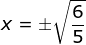Find the limit: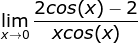The limit is infinity

To unlock this flashcard set you must be a Study.com Member.